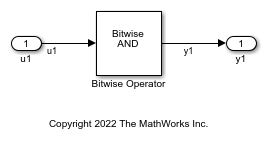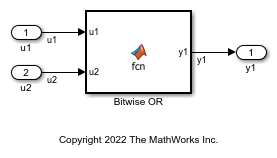Bitwise Operations

This example shows how to implement bitwise operations by using Simulink blocks, Stateflow Charts, and MATLAB Function blocks.

To include a logical operation in your model, use the Bitwise Operator block from the Logic and Bit Operations library.

1. Open example model ex_bit_logic_SL.The Logical Operator blocks perform an AND operation in the model. To change the operation, double-click the block and set the Operator field to any of the operations in the menu.

2. Double-click the block to open the Block Parameters dialog box.

3. To perform bitwise operations with a bit-mask, select Use bit mask.

If another input uses bitwise operations, clear the Use bit mask parameter and enter the number of input ports.

4. In the Bit Mask field, enter a decimal number. Use bin2dec or hex2dec to convert the input from binary or hexadecimal. In this example, enter hex2dec('D9').

5. To build the model and generate code, press Ctrl+B.

The code implementing the bitwise operator AND is in the ex_bit_logic_SL_step function in ex_bit_logic_SL.c:

/* Exported block signals */
uint8_T u1;                            /* '<Root>/u1' */
uint8_T y1;                            /* '<Root>/Bitwise Operator' */

/* Model step function */
void ex_bit_logic_SL_step(void)
{
/* S-Function (sfix_bitop): '<Root>/Bitwise Operator' incorporates:
*  Inport: '<Root>/u1'
*/
y1 = (uint8_T)(u1 & 217);
}

Stateflow Chart

1. Open example model ex_bit_logic_SF.2. Right-click the Stateflow chart to open the chart Properties.

3. Verify that the Enable C-bit operations check box is selected. For more information, see Enable C-bit operations (Stateflow).

4. To build the model and generate code, press Ctrl+B.

The code implementing the bitwise operator AND is in the ex_bit_logic_SF_step function in ex_bit_logic_SF.c:

/* Exported block signals */
uint8_T u1;                            /* '<Root>/u1' */
uint8_T y1;                            /* '<Root>/Bit_Logic' */

/* Model step function */
void ex_bit_logic_SF_step(void)
{
/* Chart: '<Root>/Bit_Logic' incorporates:
*  Inport: '<Root>/u1'
*/
y1 = (uint8_T)(u1 & 0xD9);
}

MATLAB Function Block

In this example, to show the MATLAB Function block method for implementing bitwise logic into the generated code, use the bitwise OR, '|'.

1. Open example model ex_bit_logic_ML.2. The MATLAB Function Block contains this function:

function y1 = fcn(u1, u2)
%#eml

y1 = bitor(u1, u2);
end

3. To build the model and generate code, press Ctrl+B.

The code implementing the bitwise operator OR is in the ex_bit_logic_ML_step function in ex_bit_logic_ML.c:

/* Exported block signals */
uint8_T u1;                            /* '<Root>/u1' */
uint8_T u2;                            /* '<Root>/u2' */
uint8_T y1;                            /* '<Root>/Bitwise OR' */

/* Model step function */
void ex_bit_logic_ML_step(void)
{
/* MATLAB Function: '<Root>/Bitwise OR' incorporates:
*  Inport: '<Root>/u1'
*  Inport: '<Root>/u2'
*/
y1 = (uint8_T)(u1 | u2);
}